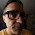## Monday, March 18, 2013

Here's a way to quickly compute the leftmost digit of a number:

```(define (leftmost-digit radix n)
(if (> radix n)
n
(let ((leftmost-digit-pair (leftmost-digit (* radix radix) n)))
(if (> radix leftmost-digit-pair)
leftmost-digit-pair
```

It's harder to compute the remaining digits.

1.Simpler, and tail recursive.

(define (leftmost-digit radix n)
(if (> radix n)
n
2.3.